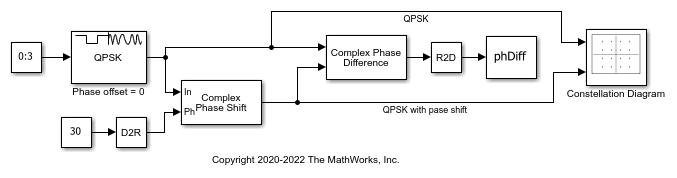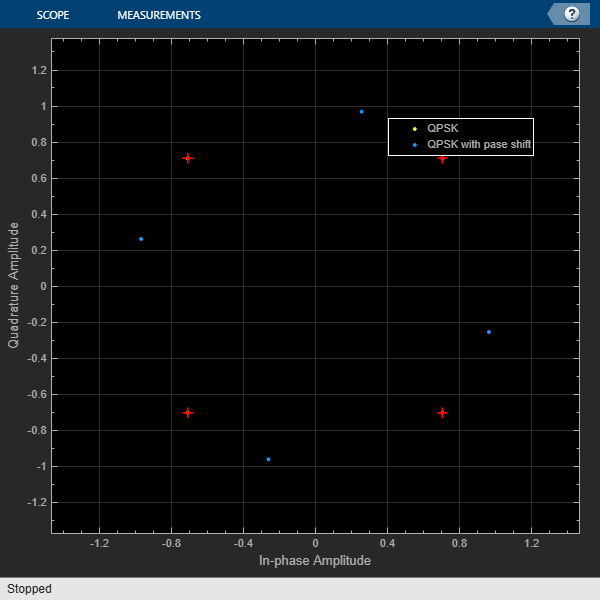Apply Complex Phase Shift to QPSK Signal

The model applies QPSK modulation, and then applies a phase shift to a signal. Calculate the phase difference and plot the constellation of the signal before and after the phase shift.

The Complex Phase Shift block applies a 30 degree phase shift to a QPSK modulated signal. The Complex Phase Difference block determines the phase difference. A To Workspace (Simulink) block to passes the calculated phase difference to the workspace. A Constellation Diagram block plots the signal constellation before and after the phase shift.The phase difference is calculated from the second input to the first input.

The calculated phase difference is: -30.0000